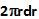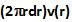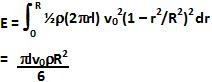## Saturday, July 18, 2009

### Irodov Problem 1.334a) Consider an infinitesimally thin cylindrical section of thickness dr of radius r around the axis. The area of cross-section of this section is. The volume of water flowing per unit time through this infinitesimally thin section is given by. The total volume of water flowing through the tube per unit time V can be found by integrating over all such cross-sections as,b) The mass of water flowing through the infinitesimally thin cross-section is given by. This entire mass of water flows at a speed v(r), hence its kinetic energy is given by. The total kinetic energy if the water in the cylinder is given by,c) Let F be the viscous force acting (and hence the driving force that is responsible for pushing the water) . The shear stress at the tube due to this force is given by. Hence we have,(note I have ignored the negative sign here since it simply gives the direction of the force).

d) The pressure difference between the ends of the pipe is what is causing the water to flow and is given by the force per unit area as,